# KR101501568B1 - video encoding, decoding apparatus and method - Google Patents

## Info

Publication number
KR101501568B1
KR101501568B1 KR1020080064903A KR20080064903A KR101501568B1 KR 101501568 B1 KR101501568 B1 KR 101501568B1 KR 1020080064903 A KR1020080064903 A KR 1020080064903A KR 20080064903 A KR20080064903 A KR 20080064903A KR 101501568 B1 KR101501568 B1 KR 101501568B1
Authority
KR
South Korea
Prior art keywords
frequency coefficients
number
frequency
before
coefficient
Prior art date
Application number
KR1020080064903A
Other languages
Korean (ko)
Other versions
KR20100004625A (en
Inventor
김하윤
김해광
전병우
이영렬
문주희
지아지에
Original Assignee
에스케이 텔레콤주식회사
Priority date (The priority date is an assumption and is not a legal conclusion. Google has not performed a legal analysis and makes no representation as to the accuracy of the date listed.)
Filing date
Publication date
Application filed by 에스케이 텔레콤주식회사 filed Critical 에스케이 텔레콤주식회사
Priority to KR1020080064903A priority Critical patent/KR101501568B1/en
Publication of KR20100004625A publication Critical patent/KR20100004625A/en
Application granted granted Critical
Publication of KR101501568B1 publication Critical patent/KR101501568B1/en

## Images

•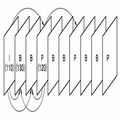•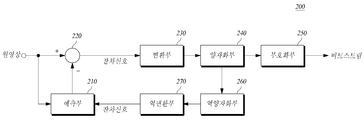•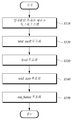•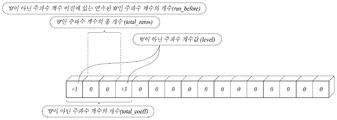••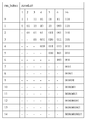•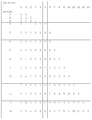•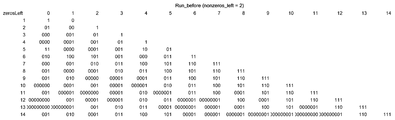•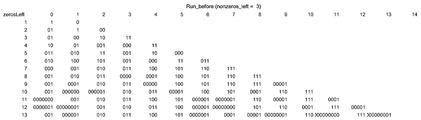••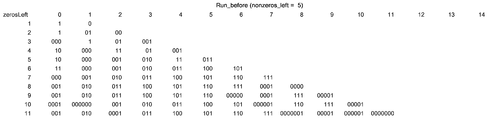•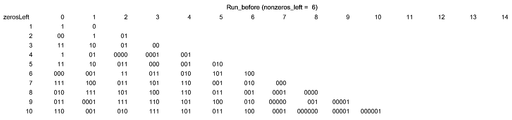•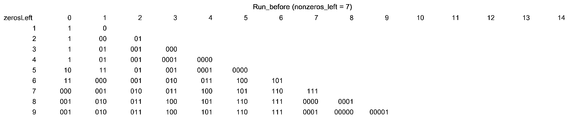•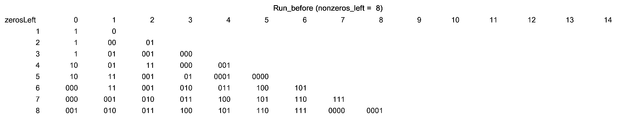•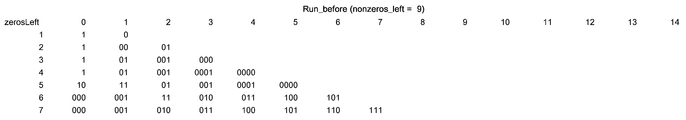•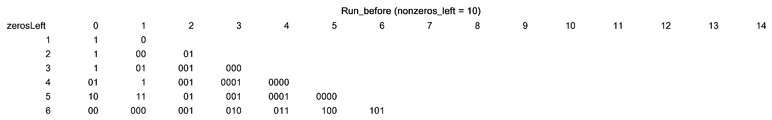•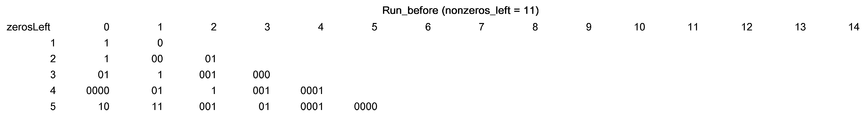•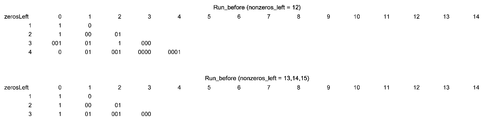•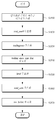•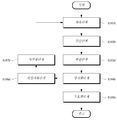•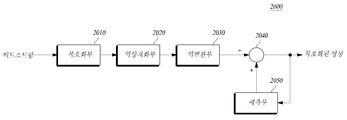•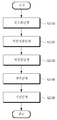•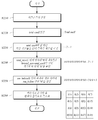## Classifications

• HELECTRICITY
• H04ELECTRIC COMMUNICATION TECHNIQUE
• H04NPICTORIAL COMMUNICATION, e.g. TELEVISION
• H04N19/00Methods or arrangements for coding, decoding, compressing or decompressing digital video signals
• H04N19/50Methods or arrangements for coding, decoding, compressing or decompressing digital video signals using predictive coding
• H04N19/503Methods or arrangements for coding, decoding, compressing or decompressing digital video signals using predictive coding involving temporal prediction
• H04N19/51Motion estimation or motion compensation
• H04N19/577Motion compensation with bidirectional frame interpolation, i.e. using B-pictures
• HELECTRICITY
• H04ELECTRIC COMMUNICATION TECHNIQUE
• H04NPICTORIAL COMMUNICATION, e.g. TELEVISION
• H04N19/00Methods or arrangements for coding, decoding, compressing or decompressing digital video signals
• H04N19/90Methods or arrangements for coding, decoding, compressing or decompressing digital video signals using coding techniques not provided for in groups H04N19/10-H04N19/85, e.g. fractals
• H04N19/93Run-length coding

## Abstract

The present invention includes a predictor for predicting a predicted pixel value of each pixel of a current block of an image and a predictor for calculating a difference between the original pixel value of each pixel of the current block and the predicted pixel value of each pixel of the current block, A quantization unit for quantizing the transformed frequency coefficients; a quantization unit for transforming the quantized frequency coefficients into one of variable length coding tables different from each other The present invention relates to an image encoding method and an image encoding method,
Image compression, coding, decoding, variable length coding, variable length conversion

## Description

TECHNICAL FIELD The present invention relates to a video encoding and decoding apparatus,

BACKGROUND OF THE INVENTION 1. Field of the Invention The present invention relates to an image encoding and decoding apparatus and method for encoding and / or decoding moving image data, and more particularly, to an apparatus and method for adaptively encoding moving image data by reflecting characteristics of an image.

Since the amount of data constituting a moving picture is much larger than that of voice or still image data, a large amount of hardware resources are required to store or transmit the data without any processing. Accordingly, in order to prevent this, the moving picture data is compressed and stored or transmitted using an encoder, and the decoder receives and reproduces the compressed moving picture data.

Moving Picture Experts Group (MPEG) and Video Coding Experts Group (VCEG) have developed superior video compression techniques superior to the existing MPEG-4 Part 2 and H.263 standards. This new standard is called H.264 / AVC (Advanced Video Coding) and is jointly announced as MPEG-4 Part 10 AVC and ITU-T Recommendation H.264.

An object of the present invention is to provide an apparatus and a method for improving the efficiency of image coding / decoding by encoding the moving picture data adaptively by reflecting characteristics of the image.

In one aspect, the present invention provides an image processing apparatus comprising: a prediction unit for predicting a predicted pixel value of each pixel of a current block of an image; A subtractor for generating a residual signal by calculating a difference value between an original pixel value of each pixel of the current block and a predicted pixel value of each pixel of the current block; A converter for converting the residual signal into frequency coefficients; A quantization unit for quantizing the transformed frequency coefficients; And quantized frequency coefficients, and when encoding run_before (the number of frequency coefficients that are '0' between a frequency coefficient that is not a current '0' and a frequency coefficient that is not a previous '0'), And an encoding unit for encoding the image using one of the tables.

According to another aspect of the present invention, there is provided a method for predicting predicted pixel values of pixels in a current block of an image, A subtraction step of calculating a difference value between an original pixel value of each pixel of the current block and a predicted pixel value of each pixel of the current block to generate a residual signal; A conversion step of converting the residual signal into frequency coefficients; A quantization step of quantizing the transformed frequency coefficients; And quantized frequency coefficients, and when encoding run_before (the number of frequency coefficients that are '0' between a frequency coefficient that is not a current '0' and a frequency coefficient that is not a previous '0'), And encoding the image using one of the tables.

According to still another aspect of the present invention, there is provided an image processing method comprising the steps of: scanning quantized frequency coefficients of each pixel of a current block of an image and arranging the quantized frequency coefficients into a one-dimensional vector; The total quantized frequency coefficients scanned are divided into TotalCoeff (number of frequency coefficients other than '0'), level (coefficient value of frequency coefficients other than '0'), total_zero ('0' (Number of frequency coefficients), run_before (the number of frequency coefficients that are '0' between a frequency coefficient that is not a current '0' and a frequency coefficient that is not a previous '0'). An encoding step of encoding the encoded data using one of the plurality of encoding methods; And outputting the encoded information as a bit stream.

According to another aspect of the present invention, there is provided an apparatus comprising: an input step of reading an input bit stream; TotalCoeff (number of frequency coefficients other than '0'), level (coefficient value of frequency coefficients other than '0'), total_zero ('0' before the last frequency coefficient, not '0' (Number of frequency coefficients), run_before (the number of frequency coefficients that are '0' between the frequency coefficient that is not the current '0' and the frequency coefficient that is not the previous '0'), Length decoding tables using one of different variable length coding tables at the time of decoding; And reconstructing the decoded frequency coefficients into an original block form.

As described above, in encoding and decoding moving picture data, the present invention can improve the efficiency of image coding / decoding by adaptively coding the characteristic of an image.

Since the moving picture is composed of 30 frames in one second, the difference between the one frame and the neighboring frame is small, so it can not be distinguished by the human eye. Therefore, if 30 frames are sprinkled in one second, the human eye recognizes that the frame is continuous.

Thus, if the previous frame and the current frame are similar, the pixel value of the next frame can be predicted from an already known pixel value constituting the previous frame (this is referred to as interprediction).

The encoding and decoding of such moving picture data is performed based on motion prediction technology. Motion prediction is performed by referring to a past frame based on a time axis or referring to both a past frame and a future frame. A frame to be referred to in encoding or decoding a current frame is referred to as a reference frame. In the block-based moving picture coding, one still picture (frame) constituting a moving picture is divided into a macro block and sub-blocks constituting a macro block, and motion is predicted on a block basis and coding is performed.

Further, the next pixel can be predicted using the correlation of pixel signals in the same frame, and the prediction error can be encoded (this is referred to as intraprediction).

In the present invention, run_before refers to the number of frequency coefficients that are '0' between a frequency coefficient that is not a current '0' and a frequency coefficient that is not a previous '0' among quantized frequency coefficients, and nonzero_left represents a current '0' TotalCoeff refers to the number of frequency coefficients that are not '0', level refers to the count value of frequency coefficients other than '0', and ' total_zero is the number of frequency coefficients that are '0' before the last frequency coefficient that is not '0'.

Also, in the present invention, trailingOnes refers to the number of frequency coefficients having an absolute value of 1 consecutively coming out last when there is a coefficient having a last continuous absolute value of 1 among zigzag scanned quantized frequency coefficients, and trailing_ones_sign_flag Refers to the sign of trailingOnes.

FIG. 1 is a diagram showing moving picture frames constituting a moving picture used in inter-picture prediction.

Referring to FIG. 1, moving picture data is composed of a series of still images. These still images are divided into GOP (Group of Picture) units. Each still image is called a frame. One GOP includes an I frame 110, a P frame 120, and a B frame. The I frame 110 is a frame that is itself encoded without using a reference frame, and the P frame 120 and the B frame 130 are frames that are encoded by performing motion estimation and compensation using a reference frame. In particular, the B frame 130 is a frame that is encoded by forward and backward (bidirectional) prediction of a past frame and a future frame, respectively.

2 is a block diagram of a moving picture encoding apparatus according to an embodiment of the present invention.

The moving picture encoding apparatus 200 includes a predictor 210, a subtractor 220, a transformer 230, a quantizer 240, and an encoder 250. [

The image encoding apparatus 300 may be a personal computer (PC), a notebook computer, a personal digital assistant (PDA), a portable multimedia player (PMP), a PlayStation Portable ), A mobile communication terminal, and the like, and may be a communication device such as a communication modem for performing communication with various devices or wired / wireless communication networks, a memory for storing various programs for encoding an image and data, a program And a microprocessor for calculating and controlling the microprocessor.

As described above, the predicting unit 210 uses one or both of inter-picture prediction based on motion prediction or intra-picture prediction in which a next pixel is predicted using the correlation of pixel signals in the same frame, A block (or a macroblock) can be predicted.

For example, the prediction unit 210 may be divided into a motion estimation unit (not shown) and a motion compensation unit (not shown). The motion estimator finds a motion prediction value of a macroblock of a current frame in a reference frame and outputs the motion difference as a motion vector. That is, the macroblock to be searched is searched in a predetermined search area of the reference frame, and the most similar macroblock is searched to output the degree of motion as a motion vector. The motion compensation unit obtains a predictive macroblock corresponding to the obtained motion vector from the reference frame.

As another example, the predicting unit 210 is an intra predictor for predicting the current macroblock of the current frame using the neighboring macroblocks of the current macroblock in the image, and may include one or more pixel values of one or more neighboring macroblocks. To predict a predictive macroblock by calculating a predicted pixel value of each pixel of the current macroblock. Here, the neighboring macroblock may be one or more surrounding macroblocks compressed before the current macroblock and located around the current macroblock.

The subtractor 220 subtracts the predictive macroblock from the macroblock of the original image frame and calculates the difference to generate a residual signal.

The conversion unit 230 converts the residual signal generated by the subtraction unit 220 into a frequency domain to obtain frequency coefficients. Here, the transform unit 230 transforms the residual signal into a frequency domain using various transform techniques for transforming a time-base image signal such as a DCT (Discrete Cosine Transform) Conversion. In the case of the I frame described with reference to FIG. 1, the transform unit 230 transforms the macroblock of the original image frame into the frequency domain.

The quantization unit 240 quantizes the frequency coefficients converted into the frequency domain by the transform unit 230.

The residual signal is obtained by subtracting the predictive macroblock from the macroblock of the original video frame, and this residual signal value is encoded to reduce the amount of data at the time of encoding. Since an error occurs in the quantization process, the motion picture data made of the bitstream includes errors generated in the conversion and quantization processes.

In order to obtain a reference frame, the moving picture encoding apparatus 200 may further include an inverse quantization unit 360 and an inverse transform unit 370. [

In order to obtain a reference frame, the quantized residual signal is combined with the predicted image from the predictor 210 via the inverse quantization unit 360 and the inverse transform unit 270, and is stored in a reference frame storage unit (not shown). In the case of the I frame, it is stored in the reference frame storage unit of the predictor 210 via the inverse quantization unit 360 and the transform unit 370. The reference image stored in the reference image storage unit is an image including an encoding error occurring in the transformation and quantization process in the original image frame.

That is, if the original image is A and the predicted image is B, the conversion unit 230 receives the A-B difference between the original image and the predicted image, and performs conversion. Then, an error component E is generated in the quantization process and the bit stream output through the encoding unit 250 is (A-B) + E. Then, the reference image storage unit stores (A-B) + E and the predicted image B, and stores the image A + E containing the error in the original image as a reference image.

The encoding unit 250 encodes the frequency coefficients quantized by the quantization unit 240 into a bit stream. As such encoding technique, entropy encoding technique may be used, but various other encoding techniques may be used without being limited thereto.

If all of the frequency coefficient values are '0', the encoding unit 250 encodes the encoded data using the coded block pattern information without entropy encoding. Otherwise, the encoding unit 250 encodes values of the frequency coefficient that are not '0' The position of the frequency coefficient.

Hereinafter, a method of encoding, for example, the case where the quantized frequency coefficients are as shown in Table 1, by the encoding unit 250 will be described.

1 (1) 0 (2) 0 (6) 0 (7) 0 (3) 0 (5) 0 (8) 0 (13) 3 (4) 0 (9) 0 (12) 0 (14) 0 (10) 0 (11) 0 (15) 0 (16)

3 is a flowchart of a method of encoding frequency coefficients quantized by the encoding unit 250 of FIG. 4 is a view showing a frequency coefficient sequence obtained by zigzag scanning the quantized frequency coefficients of FIG.

Referring to FIG. 3, the quantized frequency coefficients are scanned in a zigzag manner (S310). In Table 1, parentheses indicate the zigzag scan order. (1) 0 (2) 0 (3) 3 (4) 0 (5) 0 (6) 0 (7) 0 (8) As shown in FIG. 4, 0 (9) 0 (10) 0 (11) 0 (12) 0 (13) 0 (14) 0 (15) 0 (16).

Next, the total number of non-zero coefficients (total_coeff = 2) of quantized frequency coefficients (1, 3) other than '0' among zigzag scanned frequency coefficient columns is encoded at step S320. In step S320, total_coeff is coded using, for example, a variable length coding table of H.264 / AVC (Advanced Video Coding). At this time, when total_coeff is encoded, one of the variable length coding tables can be adaptively selected and encoded according to the situation of the surrounding macroblock.

Next, a frequency coefficient value other than '0' is encoded in a reverse or reverse order (DC direction from high frequency) to +3, +1 (S330). In step S330, the level can be encoded using, for example, a variable length coding table of H.264 / AVC (Advanced Video Coding).

Next, the number of frequency coefficients (total_zeros = 2) of '0' remaining in the DC direction in the last frequency coefficient (3 (4)) other than '0' is encoded (S340).

Next, the position of the frequency coefficient which is not '0' in the DC direction in the last frequency coefficient (3 (4)) which is not '0' is the number of frequency coefficients having a continuous value of '0' therebetween (run_before (S350). At this time, run_before according to the number (zero_left) of the frequency coefficients having the previous value of '0' remaining at the position of the frequency coefficient other than the current '0' is encoded using one of the different variable length coding tables . For example, run_before for zero_left may be encoded using different variable length coding tables according to the number of non-zero zero (0) non-zero coefficients remaining in the position of the frequency other than the current '0'.

5A and 5B are variable-length coding tables of run_before according to zero_left in a variable length coding table of H.264 / AVC.

In the run_before variable length coding table according to the zero_left in FIGS. 5A and 5B, when encoding the run_before for expressing the position of the next frequency coefficient that is not '0', the encoding length is changed based on the information theory according to zero_left have.

In this variable length coding table, for example, if zero_left is '2', run_before is encoded with lengths of 1, 2, and 2 bits in order of 0, 1, and 2, respectively. If zero_left is '3', run_before is encoded with 2 bits length for 0, 1, 2, and 3, respectively. However, this variable length coding table generally does not constitute a variable length coding table using a general probability characteristic of an image in which a frequency coefficient that is not a '0' in a low frequency portion is much higher, although the run_before becomes smaller, .

Therefore, the number of frequency coefficients non_zero_left which is not '0' existing in the DC direction of the current frequency coefficient other than '0', based on the general probability characteristic of an image in which frequency coefficients other than '0' A plurality of variable length coding tables for coding run_before according to zero_left are used.

The table value of FIG. 5A represents the number of bits used for encoding the corresponding run_before for the corresponding zero_left, and the table value of FIG. 5B represents a coding value used for encoding the corresponding run_before for the corresponding zero_left. For example, in FIG. 5A, when zero_left is' 2 'and run_before is' 2', the run_before value 2 is encoded into two bits. In FIG. 5B, when zero_left is' 2 'and run_before is' And encodes the value 2 as '00'.

FIGS. 6 through 17 illustrate different variable values encoding run_before according to zero_left for nonzero_left values of 1, 2, 3, 4, 5, 6, 7, 8, 9, 10, Length encoding tables. The variable length coding tables shown in FIGS. 6 to 17 are merely examples, and can be variously modified or modified on the basis of a general probability characteristic of an image in which a frequency coefficient other than '0' is present in a low frequency part. Since different variable length coding tables for coding run_before according to zero_left are used based on a general probability characteristic of an image in which a frequency coefficient other than '0' is low in a low frequency part, a block of a quantized frequency coefficient can be efficiently Can be encoded.

Referring to FIGS. 5A and 5B, when zero_left is 2, run_before 0, 1, and 2 are sequentially encoded into 1, 1, and 2. In contrast, when non_zero_left is 1, zero_left is 2 In this case, run_before 0, 1, and 2 are encoded in order of 2, 2, and 1 bits.

In FIG. 6, when run_before is relatively small such as 0 or 1, it is encoded into a large bit number such as 2, and when run_before is relatively large such as 2, encoding is performed with a relatively small bit number as 1, same. When non_zero_left is 1 and zero_left is 2, the total frequency coefficient existing in the DC direction at the position of the frequency coefficient other than the current '0' is 3 (1 + 2). In this case, a coefficient other than '0' existing only in one case is highly likely to be a DC frequency position due to characteristics of the image. Therefore, the three frequency coefficients after the current position (the frequency coefficient value at the current position is not '0') are most likely to be composed of frequency (DC), 0, 0> rather than <0. Therefore, since run_length = 2 in this case is the most probable, it allocates a small number of bits of 1 bit and allocates the remaining cases to 2 bits.

For the same reason, even in the variable length coding tables of FIGS. 7 to 17, the bit number of run_before may be smaller as the probability that the frequency coefficient other than the previous '0' is located before the frequency coefficient which is not '0' at present is higher.

In addition, the size of the variable length coding table can be reduced as the number of frequency coefficients (nonzero_left) that is not the previous '0' remaining is larger. That is, in the variable length coding tables of FIGS. 6 to 17, the value of nonzero_left is 1, 2, 3, 4, 5, 6, 7, 8, 9, 10, 11, 12, 13, The larger the size of the variable length coding table is. For example, when non-zero_left is 14, zero_left is 1. If nonzero_left is 15, it is not necessary to remain.

Referring again to FIG. 3, the encoded bitstreams of the quantized frequency coefficients of Table 1 through steps S310 to S350 are 2 (total_coeff), +3 (level), +1 (level) ), And 2 (run_before).

Types of information Information content Information total_coeff Number of coefficients that are not '0' 2 level Quantized coefficient value +3 level Quantized coefficient value +1 total_zeros Total number of '0' before the last coefficient 2 run_before Number of consecutive '0's before the count 2

Another example in which the encoding unit 250 encodes the quantized frequency coefficients will be described with reference to Table 3 and FIG.

15 (1) -4 (2) 9 (6) -2 (7) 7 (3) 0 (5) 0 (8) 0 (13) 0 (4) 0 (9) -1 (12) 0 (14) 1 (10) 0 (11) 0 (15) 0 (16)

FIG. 18 is a flowchart of another method of encoding the quantized frequency coefficients in the encoding unit of FIG. 2; 3 are the same as those described with reference to FIG. 3, and therefore, will be described briefly.

Referring to FIG. 18, first, quantized frequency coefficients are zigzag scanned (S1810). (3) 0 (4) 0 (5) 9 (6) -2 (7) 0 (8) The frequency coefficient columns obtained by zigzag scanning the quantized frequency coefficients in Table 3 are 15 0 (9) 1 (10) 0 (11) -1 (12) 0 (13) 0 (14) 0 (15) 0 (16).

Next, the number of quantized frequency coefficients (15, -4, 7, 9, -2, 1, -1) other than '0' (total_coeff = 7) is encoded (S12020).

(Trailing_ones_sign_flag = -, trailing_ones_sign_flag = -), where the absolute value is 1 and the absolute value is 1, +) Are encoded in the reverse order (S12030, S12040). In steps S12030 and S12040, trailingones and trailing_ones_sign_flag can be encoded using, for example, a variable length coding table of H.264 / AVC.

Next, the frequency coefficient values (levels) other than '0' except coefficients having an absolute value of 1 are encoded in the reverse order (high frequency to DC direction) as -2, +9, +7, -4 and +15 S12050).

Next, the number of frequency coefficients (total_zeros = 5) of '0' remaining in the DC direction in the last frequency coefficient (-1 (12)) other than '0' is encoded (S1860).

Next, the position of the frequency coefficient which is not '0' in the DC direction in the last frequency coefficient (-1 (12)) which is not '0' is referred to as the number of frequency coefficients run_before (Step S1870). At this time, run_before is coded in the reverse order so as to correspond to a frequency coefficient value (level) rather than '0' by using the variable length coding tables of FIGS. 6 to 17. That is, run_before between -1 (12) and +1 (10) is 1, run_before between +1 (10) and -2 (7) is 2, and between -2 (7) and +9 Run_before of run_before between +9 (6) and +7 (3) is 2, between +7 (3) and -4 (2) The reverse order is 1, 2, 0, 2, 0, 0. Each of the run_before (1, 2, 0, 2, 0, 0) selects one of the variable length coding tables of FIGS. 6 to 17 in consideration of nonzero_lefts, and encodes Value is determined.

Table 4 shows the bitstreams in which the quantized frequency coefficients of Table 3 are encoded through steps S1810 to S1870. That is, the bitstreams are 7 (total_coeff), 2 (trailingones), - (trailing_ones_sign_flag), + (trailing_ones_sign_flag), -2 (level), +9 (level) (Run_before), 0 (run_before), 0 (run_before), and 0 (run_before) information in the order of 15 (level), 5 (total_zeros), 1 (run_before), 2

Types of information Information total_coeff 7 trailingones 2 trailing_ones_sign_flag - trailing_ones_sign_flag + level -2 level +9 level +7 level -4 level +15 total_zeros 5 run_before One run_before 2 run_before 0 run_before 2 run_before 0 run_before 0

As described above, the image encoded with the bit stream by the image encoding apparatus 200 can be transmitted in real time or in non-real time through a wired / wireless communication network such as the Internet, a local area wireless communication network, a wireless LAN network, a WiBro network, And transmitted to a video decoding device through a communication interface such as a serial bus (USB: Universal Serial Bus), decoded by the video decoding device, and restored and reproduced as an image.

19 is a flowchart of an image encoding method according to another embodiment of the present invention.

Referring to FIG. 19, the image encoding method according to another embodiment of the present invention includes a prediction step S1910, a subtraction step S1920, a conversion step S1930, a quantization step S1940, and an encoding step S1950 .

The prediction step S1910 is a step of predicting the pixel value of each pixel of the current block of the image. The prediction step S1910 generates a prediction block by predicting the pixel value of each pixel of the current block by a previous block of the previous frame or a neighboring block of the current frame.

In the subtraction step S1920, the difference between the original pixel value of the current block to be encoded and the predicted pixel value of the predictive block (i.e., residual signal) is calculated in order to reduce the amount of encoded data and increase the coding efficiency.

The converting step S1930 is a step of converting the generated residual signal into a frequency domain using a DCT transform or the like.

The quantization step S1940 is a step of quantizing the frequency coefficients converted into the frequency domain.

The coding step S1950 is a step of coding the quantized frequency coefficients into the types of information of Table 2 or Table 4 using the variable length coding table. The concrete steps of the encoding step S1950 have been described in detail with reference to the encoding method described with reference to FIG. 3 to FIG.

The method of coding the quantized frequency coefficients in a context-based manner using the variable length coding tables in the encoding step S1950 is referred to as context adaptive variable length coding (CAVLC). Embodiments of the present invention are based on context-based adaptive variable length coding in the step of coding quantized frequency coefficients.

Referring to FIG. 19, the image encoding method according to another embodiment of the present invention may further include an inverse quantization step (S1960) and an inverse transformation step (S1970).

The inverse quantization step S1960 and the inverse transformation step S1970 are steps of generating a residual signal through inverse quantization and inverse transformation of the quantized frequency coefficients. That is, the quantized residual signal is combined with the predicted image in the prediction step S1910 through the inverse quantization step S1960 and the inverse transformation step S1970, and is stored as a reference frame.

As described above, the image encoded with the bit stream by the image encoding apparatus 200 can be transmitted in real time or in non-real time through a wired / wireless communication network such as the Internet, a local area wireless communication network, a wireless LAN network, a WiBro network, And transmitted to a video decoding device through a communication interface such as a serial bus (USB: Universal Serial Bus), decoded by the video decoding device, and restored and reproduced as an image.

20 is a block diagram schematically showing an electronic configuration of an image decoding apparatus according to another embodiment of the present invention.

The video decoding apparatus 2000 according to another embodiment of the present invention predicts a current block of a video using one or more neighboring blocks around the current block and decodes the video, An inverse quantization unit 2020, an inverse transformation unit 2030, an addition unit 2040, and a prediction unit 2050.

The image decoding apparatus 800 may be implemented by a computer such as a personal computer (PC), a notebook computer, a personal digital assistant (PDA), a portable multimedia player A portable communication terminal, a PMP (Portable Multimedia Player), a PlayStation Portable (PSP), a mobile communication terminal, and the like. The communication device such as a communication modem for performing communication with various devices or wired / wireless communication networks, A memory for storing various programs and data for performing the functions, a microprocessor for executing and controlling the programs, and the like.

The decoding unit 2010 decodes the bitstream and extracts the quantized frequency coefficients. That is, the decoding unit 2010 decodes a bitstream, which is an image encoded by the image encoding apparatus 300, and extracts quantized frequency coefficients including pixel information of a current block of the image.

The decoding unit 2010 decodes quantized frequency coefficients using the variable length coding tables described above from the information included in Table 2 or Table 4 included in the bitstream. For example, the decoding unit 2010 decodes encoded information 2 (total_coeff), +3 (level), +1 (level), 2 (total_zeros), 2 (1) 0 (2) 0 (3) 3 (4) 0 (5) shown in FIG. 4 using the variable length coding tables shown in FIGS. 6 to 17, 0) (14) 0 (15) 0 (16)), and then decodes it into the next Into blocks of the number of quantized frequencies shown in Table 1. &lt; tb &gt; &lt; TABLE &gt; A concrete decoding method will be described with reference to FIGS. 21 and 22. FIG.

The inverse quantization unit 2020 dequantizes the frequency coefficients extracted from the bit stream by the decoding unit 2010. [

The inverse transform unit 2030 inverse transforms the frequency coefficients inversely quantized by the inverse quantization unit 2020 into a time domain to generate a residual signal.

The adder 2030 restores the original pixel value of the current block by adding the predicted pixel value of each pixel of the current block predicted by the predictor 2050 with the residual signal inversely transformed by the inverse transform unit 2020. [

FIG. 21 is a flowchart illustrating a video decoding method according to another embodiment of the present invention.

The video decoding apparatus 800 receives and stores a bitstream of an image through a wired / wireless communication network or a cable. In order to reproduce an image according to a user's selection or an algorithm of another program being executed, Prediction is performed using one or more neighboring blocks around the current block or the current block, and the image is decoded and restored.

To this end, a video decoding method according to another embodiment of the present invention restores information contained in a bitstream to a quantized frequency coefficient indicating information on a pixel value of a current block of an image using a variable length coding table An inverse quantization step (S2120) of inverse quantizing the extracted quantized frequency coefficients; an inverse transform step (S2130) of inversely transforming the inversely quantized frequency coefficients into a time domain to restore a residual signal; (S2140) of predicting the current block using the current block indicated by the residual signal and one or more neighboring blocks around the current block, in step S2140. In step S2130, the residual signal of the inverse- An addition step S2 (S2) of adding the predicted pixel value of each pixel of the current block predicted in step S2140 and restoring the original pixel value of the current block, 150).

22 is a flowchart of a decoding method for decoding a block of frequency coefficients from information of a bitstream encoded in the decoding step of FIG.

Referring to FIG. 22, a decoding method according to another embodiment of the present invention includes first decoding the frequency coefficients shown in Table 2 using the bitstream information encoded with the encoding method described with reference to FIGS. 3 through 17 (total_coeff), +3 (level), +1 (level), 2 (total_zeros), 2 (run_before).

Next, the first information 2 (total_coeff) is read (S2220), and the next information (+3 (level), +1 (level)) is read by the total_coeff value (S2230). The frequency coefficient read in step S2230 is +3, +1.

Next, the next information 2 (total_zeros) is read and the coefficients of '0' are added to the frequency coefficients obtained in step S2230 by 16-total_zeros-total_coeff = 12 (S2240). The frequency coefficient column obtained in step S2240 is 0 0 0 0 0 0 0 0 0 0 0 +3 +1.

Next, the next information (2) (run_before) is read and a coefficient of '0' is added as a run_before value between the frequency coefficients obtained in step S2240 (S2250). The frequency coefficient column obtained in step S2250 is 0 0 0 0 0 0 0 0 0 0 0 +3 +3 0 +1. At this time, since run_before is coded by the variable length coding table of FIG. 6, the run_before value may be decoded using the variable length coding table of FIG. 6 in step S2250, and then step S2260 may be performed.

Next, the frequency coefficient sequences obtained in step S2250 are outputted in reverse order, and then the original frequency coefficients are restored to the original block form as shown in Table 2 (S2260).

The quantized frequency coefficient sequence shown in Table 2 has been described above with reference to FIG. 22, but the quantized frequency coefficient sequence shown in Table 4 can also be decoded in the same way. However, since the number of frequency coefficients having an absolute value of 1 and their sign values are included, the original frequency coefficient sequence and the original macroblock can be restored by using these information and the values of run_before, total_coeff, and total_zeros.

Since the information of the bit stream described above is coded using the variable-length coding table by the context-based adaptive variable length coding, the variable length coding table is used in the decoding step to decode the quantized frequency coefficients, It can be restored to a block.

On the other hand, the moving picture coding method and the decoding method described above can be written in a computer program. The code and code segments that make up the program can be easily deduced by a computer programmer in the field. In addition, the program is stored in a computer-readable medium and readable and executed by a computer to implement a video encoding and decoding method. The information storage medium includes a magnetic recording medium, an optical recording medium, and a carrier wave medium.

The foregoing description is merely illustrative of the technical idea of the present invention, and various changes and modifications may be made by those skilled in the art without departing from the essential characteristics of the present invention. Therefore, the embodiments disclosed in the present invention are intended to illustrate rather than limit the scope of the present invention, and the scope of the technical idea of the present invention is not limited by these embodiments. The scope of protection of the present invention should be construed according to the following claims, and all technical ideas within the scope of equivalents should be construed as falling within the scope of the present invention.

FIG. 1 is a diagram showing moving picture frames constituting a moving picture used in inter-picture prediction.

2 is a block diagram of a moving picture encoding apparatus according to an embodiment of the present invention.

3 is a flowchart of a method of encoding frequency coefficients quantized by the encoding unit of FIG.

FIG. 4 is a view showing a frequency coefficient sequence obtained by zigzag scanning the quantized frequency coefficients of FIG. 3. FIG.

5A and 5B are variable-length coding tables of run_before according to zero_left among variable length coding tables of H.264 / AVC (Advanced Video Coding).

FIGS. 6 through 17 illustrate different variable values encoding run_before according to zero_left for nonzero_left values of 1, 2, 3, 4, 5, 6, 7, 8, 9, 10, Length encoding tables.

FIG. 18 is a flowchart of another method of encoding the quantized frequency coefficients in the encoding unit of FIG. 2;

19 is a flowchart of an image encoding method according to another embodiment of the present invention.

20 is a block diagram schematically showing an electronic configuration of an image decoding apparatus according to another embodiment of the present invention.

FIG. 21 is a flowchart illustrating a video decoding method according to another embodiment of the present invention.

22 is a flowchart of a decoding method for decoding a block of frequency coefficients from information of a bitstream encoded in the decoding step of FIG.

## Claims (25)

1. A predictor for predicting a predicted pixel value of each pixel of a current block of an image;
A subtractor for generating a residual signal by calculating a difference value between an original pixel value of each pixel of the current block and a predicted pixel value of each pixel of the current block;
A converter for converting the residual signal into frequency coefficients;
A quantizer for quantizing the transformed frequency coefficients; And
Encoding the quantized frequency coefficients, encoding the quantized frequency coefficients, non_zero_left (current &quot; 0 &quot;) when encoding run_before (the number of frequency coefficients that are '0' 0 &quot; in the DC direction using one of the different variable length coding tables based on the remaining number of frequency coefficients that are not &quot; 0 &quot; And an encoding unit
And an image encoding device.
2. delete
3. The method according to claim 1,
The encoding unit may convert the quantized frequency coefficients into a sum of the total number of frequency coefficients before Total Coeff (number of frequency coefficients other than 0), level (coefficient value of frequency coefficients other than 0), total_zero And a number of frequency coefficients of ' 0 ') in the context-based adaptive variable length coding.
4. The method according to claim 1,
In the variable length coding table, the number of bits of the run_before is smaller as the probability that the frequency coefficient that is not the previous '0' is located before the frequency coefficient that is not the current '0'.
5. The method according to claim 1,
Wherein the variable length coding table has a smaller size as the value of non_zero_left increases.
6. The method of claim 3,
Wherein the encoding unit encodes the quantized frequency coefficients scanned in a zigzag manner in the TotalCoeff, the level, the total_zero, and the run_before order.
7. The method according to claim 6,
The encoding unit may include a trailingOnes (the number of frequency coefficients having an absolute value of 1 successively coming out) and a trailing_ones_sign_flag (when the number of consecutive frequency coefficients is 1) when there is a coefficient having a last consecutive absolute value of the quantized frequency coefficients scanned in zigzags. code of trailingOnes) is further included,
Wherein the trailingOnes and the trailing_ones_sign_flag are located between the TotalCoeff and the level.
8. A prediction step of predicting a predicted pixel value of each pixel of a current block of an image;
A subtraction step of calculating a difference value between an original pixel value of each pixel of the current block and a predictive pixel value of each pixel of the current block to generate a residual signal;
A conversion step of converting the residual signal into frequency coefficients;
A quantization step of quantizing the transformed frequency coefficients; And
Encoding the quantized frequency coefficients, encoding the quantized frequency coefficients, non_zero_left (current &quot; 0 &quot;) when encoding run_before (the number of frequency coefficients that are '0' 0 &quot; in the DC direction using one of the different variable length coding tables based on the remaining number of frequency coefficients that are not &quot; 0 &quot; Encoding step
Wherein the image encoding method comprises:
9. delete
10. 9. The method of claim 8,
Wherein the encoding step comprises the steps of: converting the quantized frequency coefficients into TotalCoeff (number of frequency coefficients other than '0'), level (coefficient value of frequency coefficients other than '0'), total_zero The number of frequency coefficients of '0' in the context-based adaptive variable length coding).
11. 9. The method of claim 8,
Wherein in the variable length coding table, the number of bits of the run_before is smaller the higher the probability that the frequency coefficient that is not the previous '0' is located before the frequency coefficient that is not the current '0'.
12. 9. The method of claim 8,
Wherein the variable length coding table is reduced in size as the value of nonzero_left increases.
13. 11. The method of claim 10,
Wherein the encoding step encodes the quantized frequency coefficients scanned by zigzag in the order of TotalCoeff, level, total_zero, and run_before.
14. 14. The method of claim 13,
Wherein the coding step includes: trailingOnes (the number of frequency coefficients having an absolute value of 1 successively coming out) and trailing_ones_sign_flag (1) when there is a coefficient having a last continuous absolute value of the quantized frequency coefficients scanned in zigzags The code of the trailingOnes)
Wherein the trailingOnes and the trailing_ones_sign_flag are located between the TotalCoeff and the level.
15. A scanning step of scanning the quantized frequency coefficients of each pixel of the current block of the image into a one-dimensional vector;
The scanned quantized frequency coefficients are divided into TotalCoeff (number of frequency coefficients other than '0'), level (coefficient value of frequency coefficients other than '0'), total_zero ('0' , And run_before (the number of frequency coefficients that are '0' between the frequency coefficient that is not the current '0' and the frequency coefficient that is not the previous '0') in the order of nonzero_left 0 &quot;) using one of the different variable length coding tables based on the remaining number of non-zero frequency coefficients remaining at the position of the frequency coefficient other than the current '0' A coding step of coding the video data in the direction of the video data;
And outputting the encoded information as a bit stream
And a decoding step of decoding the encoded data.
16. delete
17. 16. The method of claim 15,
In the variable length coding table, the number of bits of the run_before is smaller as the probability that the frequency coefficient that is not the previous '0' is located before the frequency coefficient that is not the current '0'.
18. 16. The method of claim 15,
Wherein the variable length coding table is reduced in size as the value of nonzero_left increases.
19. 16. The method of claim 15,
If there is a frequency coefficient having the last consecutive absolute value of 1 among the quantized frequency coefficients scanned zigzag, a trailingOnes (the number of frequency coefficients having an absolute value of 1 successively coming out) and a trailing_ones_sign_flag ) Are encoded,
Wherein the trailingOnes and the trailing_ones_sign_flag are located between the TotalCoeff and the level.
20. 16. The method of claim 15,
Wherein in the encoding step, if the level and the run_before are plural, the level and the run_before are encoded in the DC direction in the last frequency coefficient that is not '0'.
21. An input step of reading an input bit stream;
TotalCoeff (number of frequency coefficients other than '0'), level (coefficient value of frequency coefficients other than '0'), total_zero ('0' before the last frequency coefficient which is not '0' '), Decode the frequency coefficients using run_before (the number of frequency coefficients that are' 0 'between the frequency coefficient that is not the current' 0 'and the frequency coefficient that is not the previous' 0'), and the run_before Decoded using one of different variable length coding tables based on non_zero_left (the number of frequency coefficients that are not the previous '0' remaining at the position of the frequency coefficient other than the current '0') at the time of decoding Wow;
And reconstructing the decoded frequency coefficients into an original block form.
22. delete
23. 22. The method of claim 21,
In the variable length coding table, the number of bits of the run_before is smaller as the probability that the frequency coefficient that is not the previous '0' is located before the frequency coefficient that is not the current '0' is higher.
24. 22. The method of claim 21,
Wherein the variable length coding table is reduced in size as the value of nonzero_left increases.
25. 22. The method of claim 21,
In the input step, the input bitstream information includes trailingOnes (the number of frequency coefficients having an absolute value of 1 consecutively coming out) and trailing_ones_sign_flag the sign of the trailingOnes) is further included between the TotalCoeff and the level,
Wherein the decoding step decodes the frequency coefficients by further using the trailingOnes and the trailing_ones_sign_flag.
KR1020080064903A 2008-07-04 2008-07-04 video encoding, decoding apparatus and method KR101501568B1 (en)

## Priority Applications (1)

Application Number Priority Date Filing Date Title
KR1020080064903A KR101501568B1 (en) 2008-07-04 2008-07-04 video encoding, decoding apparatus and method

## Applications Claiming Priority (3)

Application Number Priority Date Filing Date Title
KR1020080064903A KR101501568B1 (en) 2008-07-04 2008-07-04 video encoding, decoding apparatus and method
US13/002,582 US9319710B2 (en) 2008-07-04 2009-06-26 Video encoding and decoding apparatus and method
PCT/KR2009/003457 WO2010002148A2 (en) 2008-07-04 2009-06-26 Video encoding and decoding apparatus and method

## Publications (2)

Publication Number Publication Date
KR20100004625A KR20100004625A (en) 2010-01-13
KR101501568B1 true KR101501568B1 (en) 2015-03-12

# Family

## Family Applications (1)

Application Number Title Priority Date Filing Date
KR1020080064903A KR101501568B1 (en) 2008-07-04 2008-07-04 video encoding, decoding apparatus and method

## Country Status (3)

US (1) US9319710B2 (en)
KR (1) KR101501568B1 (en)
WO (1) WO2010002148A2 (en)

## Families Citing this family (3)

* Cited by examiner, † Cited by third party
Publication number Priority date Publication date Assignee Title
KR20130049526A (en) * 2011-11-04 2013-05-14 오수미 Method for generating reconstructed block
KR20130049523A (en) 2011-11-04 2013-05-14 오수미 Apparatus for generating intra prediction block
KR20130050404A (en) * 2011-11-07 2013-05-16 오수미 Method for generating reconstructed block in inter prediction mode

## Citations (4)

* Cited by examiner, † Cited by third party
Publication number Priority date Publication date Assignee Title
US20070110161A1 (en) * 2005-11-02 2007-05-17 Katsuo Saigo Motion vector estimation apparatus
WO2008013020A1 (en) * 2006-07-26 2008-01-31 Sony Corporation Decoding method, program for decoding method, recording media with recorded program for decoding method, and decoding device
KR100801967B1 (en) * 2006-07-07 2008-02-12 광주과학기술원 Encoder and decoder for Context-based Adaptive Variable Length Coding, methods for encoding and decoding the same, and a moving picture transmission system using the same
US20080074296A1 (en) * 2001-11-22 2008-03-27 Shinya Kadono Variable length coding method and variable length decoding method

## Family Cites Families (27)

* Cited by examiner, † Cited by third party
Publication number Priority date Publication date Assignee Title
US6104754A (en) * 1995-03-15 2000-08-15 Kabushiki Kaisha Toshiba Moving picture coding and/or decoding systems, and variable-length coding and/or decoding system
US8848787B2 (en) * 2007-10-15 2014-09-30 Qualcomm Incorporated Enhancement layer coding for scalable video coding
US20040120404A1 (en) * 2002-11-27 2004-06-24 Takayuki Sugahara Variable length data encoding method, variable length data encoding apparatus, variable length encoded data decoding method, and variable length encoded data decoding apparatus
CN1639984B (en) * 2002-03-08 2011-05-11 日本电信电话株式会社 Digital signal encoding method, decoding method, encoding device, decoding device
US7099387B2 (en) 2002-03-22 2006-08-29 Realnetorks, Inc. Context-adaptive VLC video transform coefficients encoding/decoding methods and apparatuses
US6795584B2 (en) * 2002-10-03 2004-09-21 Nokia Corporation Context-based adaptive variable length coding for adaptive block transforms
US6646578B1 (en) * 2002-11-22 2003-11-11 Ub Video Inc. Context adaptive variable length decoding system and method
KR100959532B1 (en) * 2003-12-18 2010-05-27 엘지전자 주식회사 Decoding method of CAVLC
US20050259742A1 (en) * 2004-05-21 2005-11-24 Hellman Timothy M System and method for choosing tables in CAVLC
US7830964B2 (en) * 2004-11-24 2010-11-09 Lsi Corporation Method and/or apparatus for parsing compressed video bitstreams
JP4502384B2 (en) * 2004-11-25 2010-07-14 キヤノン株式会社 Variable length code decoding apparatus and variable length code decoding method
US20060126744A1 (en) * 2004-12-10 2006-06-15 Liang Peng Two pass architecture for H.264 CABAC decoding process
JP4271134B2 (en) * 2004-12-10 2009-06-03 株式会社東芝 Variable length coding decoder and decoding method
KR100674941B1 (en) 2005-01-13 2007-01-26 삼성전자주식회사 Context adaptive variable length coding apparatus and method thereof
JP2006203682A (en) * 2005-01-21 2006-08-03 Nec Corp Converting device of compression encoding bit stream for moving image at syntax level and moving image communication system
US7751636B2 (en) * 2005-09-23 2010-07-06 Faraday Technology Corp. Method for decoding transform coefficients corresponding to an image
US7245242B2 (en) * 2005-11-28 2007-07-17 Conexant Systems, Inc. Decoding systems and methods
JP4844449B2 (en) * 2006-04-17 2011-12-28 日本ビクター株式会社 Moving picture encoding apparatus, method, program, moving picture decoding apparatus, method, and program
KR100813877B1 (en) * 2006-05-11 2008-03-18 전자부품연구원 Effective decoding method of h.264/avc context-based adaptive variable length coding
TWI314820B (en) * 2006-05-26 2009-09-11 Ind Tech Res Inst Pattern-search based method for context-adaptive variable length coding/decoding
US8325819B2 (en) * 2006-10-12 2012-12-04 Qualcomm Incorporated Variable length coding table selection based on video block type for refinement coefficient coding
JP4356028B2 (en) * 2007-05-17 2009-11-04 ソニー株式会社 Information processing apparatus and method
BRPI0818444A2 (en) * 2007-10-12 2016-10-11 Qualcomm Inc adaptive encoding of video block header information
US8938009B2 (en) * 2007-10-12 2015-01-20 Qualcomm Incorporated Layered encoded bitstream structure
US20100260258A1 (en) * 2007-12-13 2010-10-14 Kyoko Ueda Image coding device, coding rate conversion device, audio video stream recording device, network distribution system, coefficient conversion device, integrated circuit, and image coding method
JP2010278668A (en) * 2009-05-27 2010-12-09 Sony Corp Coding apparatus and method, and decoding apparatus and method
US8410959B2 (en) * 2010-04-09 2013-04-02 Qualcomm, Incorporated Variable length codes for coding of video data

## Patent Citations (4)

* Cited by examiner, † Cited by third party
Publication number Priority date Publication date Assignee Title
US20080074296A1 (en) * 2001-11-22 2008-03-27 Shinya Kadono Variable length coding method and variable length decoding method
US20070110161A1 (en) * 2005-11-02 2007-05-17 Katsuo Saigo Motion vector estimation apparatus
KR100801967B1 (en) * 2006-07-07 2008-02-12 광주과학기술원 Encoder and decoder for Context-based Adaptive Variable Length Coding, methods for encoding and decoding the same, and a moving picture transmission system using the same
WO2008013020A1 (en) * 2006-07-26 2008-01-31 Sony Corporation Decoding method, program for decoding method, recording media with recorded program for decoding method, and decoding device

## Also Published As

Publication number Publication date
US9319710B2 (en) 2016-04-19
KR20100004625A (en) 2010-01-13
WO2010002148A2 (en) 2010-01-07
US20110176605A1 (en) 2011-07-21
WO2010002148A3 (en) 2010-04-22

## Similar Documents

Publication Publication Date Title
CN1200568C (en) Optimum scanning method for change coefficient in coding/decoding image and video
JP4902642B2 (en) System and method for scalable encoding and decoding of multimedia data using multiple layers
KR101431545B1 (en) Method and apparatus for Video encoding and decoding
KR100813963B1 (en) Method and apparatus for loseless encoding and decoding image
KR20090058954A (en) Video coding method and apparatus using side matching, and video decoding method and appartus thereof
KR101458471B1 (en) Method and Apparatus for Encoding and Decoding Vedio
KR20130095311A (en) Video coding using function-based scan order for transform coefficients
JP4393875B2 (en) System and method for providing a single layer video encoded bitstream suitable for reduced complexity decoding
JP6193446B2 (en) Coefficient scanning in video coding.
KR20110047697A (en) Method and apparatus for encoding and decoding coding unit of picture boundary
KR101365567B1 (en) Method and apparatus for prediction video encoding, and method and apparatus for prediction video decoding
KR20130016172A (en) Decoding method of inter coded moving picture
KR20130105894A (en) Mode dependent scanning of coefficients of a block of video data
KR101082233B1 (en) Picture Coding Method Picture Decoding Method Picture Coding Apparatus Picture Decoding Apparatus and Program thereof
US8260069B2 (en) Color image encoding and decoding method and apparatus using a correlation between chrominance components
JP4491349B2 (en) Intra-coding method and apparatus for video data
KR101732767B1 (en) Quantized pulse code modulation in video coding
DK2727353T3 (en) Video Coding using adaptive bevægelsesvektoropløsning
KR101379185B1 (en) Prediction Mode Selection Method and Apparatus and Video Enoding/Decoding Method and Apparatus Using Same
BRPI0214328B1 (en) method for decompressing video data, and video decoder for decoding video frames
JP6025726B2 (en) Video decoding device
KR20090097688A (en) Method and apparatus of encoding/decoding image based on intra prediction
KR101527087B1 (en) Video decoding method and computer readable redording meduim thereof
JP4927207B2 (en) Encoding method, decoding method and apparatus
KR101379188B1 (en) Video Coding and Decoding Method and Apparatus for Macroblock Including Intra and Inter Blocks

## Legal Events

Date Code Title Description
A201 Request for examination
E902 Notification of reason for refusal
E902 Notification of reason for refusal
E902 Notification of reason for refusal
E90F Notification of reason for final refusal
E701 Decision to grant or registration of patent right
GRNT Written decision to grant
FPAY Annual fee payment

Payment date: 20180305

Year of fee payment: 4

FPAY Annual fee payment

Payment date: 20190304

Year of fee payment: 5